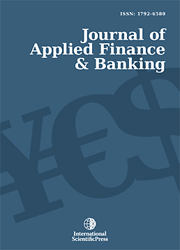# Journal of Applied Finance & Banking

#### Integral Equation Methods for Pricing Perpetual Bermudan Options

•[ Download ]
• Times downloaded: 2560
• Abstract

This paper develops integral equation methods to the pricing problems of perpetual Bermudan options. By mathematical derivation, the optimal exercise boundary of perpetual Bermudan options can be determined by an integral-form nonlinear equation which can be solved by a root-finding algorithm. With the computational value of optimal exercise, the price of perpetual Bermudan options is written by a Fredholm integral equation. A collocation method is proposed to solve the Fredholm integral equation and the price of the options is thus computed. Numerical examples are provided to show the reliability of the method, verify the validity of replacing the early exercise policies with perpetual American options, and explore a simplified computational process using the formulas for perpetual American options.ISSN: 1792-6599 (Online)
1792-6580 (Print)